# Precalculus : Express Logarithms in Expanded Form

## Example Questions

← Previous 1 2 Next →

### Example Question #81 : Exponential And Logarithmic Functions

Completely expand this logarithm: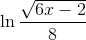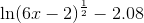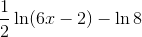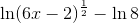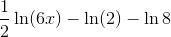Explanation:

We expand logarithms using the same rules that we use to condense them.

Here we will use the quotient property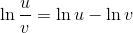and the power property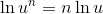Use the quotient property: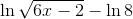Now use the power property:### Example Question #12 : Express Logarithms In Expanded Form

Expand the logarithm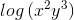Explanation:

In order to expand the logarithmic expression, we use the following properties

•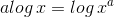•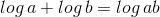As such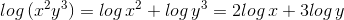← Previous 1 2 Next →

### All Precalculus Resources